# How to Find the Area and Perimeter of a Semicircle

Laura Foist, Joseph Vigil, Matthew Bergstresser
• Author
Laura Foist

Laura has a Masters of Science in Food Science and Human Nutrition and has taught college Science.

• Instructor
Joseph Vigil

Joseph has a master's degree in literature as well as alternative teaching and ESL educator certifications. He has worked with middle school, high school, and college students in writing and language arts.

• Expert Contributor
Matthew Bergstresser

Matthew has a Master of Arts degree in Physics Education. He has taught high school chemistry and physics for 14 years.

What is a semicircle? Learn the area of a semicircle formula and perimeter of a semicircle formula. See worked examples of how to find area and perimeter. Updated: 07/20/2021

Show

## What is a Semicircle?

A circle is a round shape, with no corners, where all radii (distance from the edge to the center) is the same. A semicircle takes the circle and cuts it in half through the diameter (a line that goes from one edge of the circle to the other side and passes through the center of the circle), in other words it is half of a circle. A semicircle can be closed off with a line, creating a semicircle shape, or it can be left with no line, creating a semicircle arc. Typically when referring to a semicircle the, shape with the line closing the bottom is being referenced.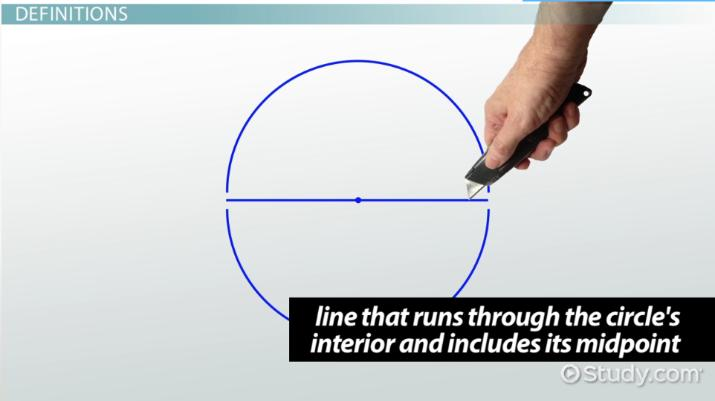An error occurred trying to load this video.

Try refreshing the page, or contact customer support.

Coming up next: Perimeter and Area of a Semicircle Problems & Flashcards

### You're on a roll. Keep up the good work!

Replay
Your next lesson will play in 10 seconds
• 0:01 Definitions
• 0:44 Area of a Semicircle
• 2:55 Perimeter of a Semicircle
• 5:14 Lesson Summary
Save Save

Want to watch this again later?

Timeline
Autoplay
Autoplay
Speed Speed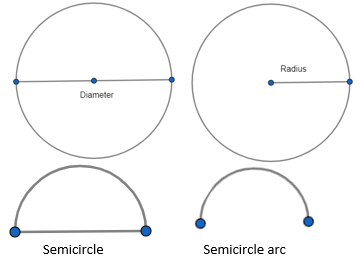## Area of a Semicircle

The area of a semicircle refers to the amount of area inside of the lines of the semicircle shape. The semicircle arc has no area since it is not closed off into a shape. Area is given in units that are 'squared', such as square inches or square meters. The term 'squared' comes from how formulas for area is derived, based on how many individual square shapes can fit inside the lines of the shape.

### Area of a Semicircle Formula

To find the area of a circle, the formula is pi times the radius squared. Since a semicircle is half of a circle, the area of a semicircle is half of a circle. So the formula to find the area of a semicircle is pi times radius squared divided by 2, or pi times diameter squared divided by 8: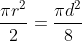In this formula r refers to the radius of the semicircle (or the radius of the corresponding circle), and d refers to the diameter. Either formula can be used depending on what information is provided.

### How to Find the Area of a Semicircle

Let's first find the area of a semicircle when given the radius: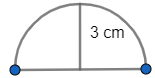In this semicircle, the radius is equal to 3 cm. Put '3' in for r in the formula to find the area of a semicircle with a radius of 3 cm.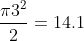So the area of this semicircle is 14.1 cm2

Now let's find the area of a semicircle from the diameter: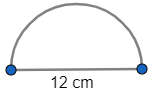The diameter is equal to 12 cm, so put in '12' for d in the formula: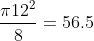This semicircle has an area of 56.5 cm2.

## Perimeter of Semicircle

The perimeter refers to the length of the line going around the entire shape. It is how long the line would be if the line was laid out in one straight line. It is the distance to walk around the entire shape. The perimeter of a semicircle is equal to the length of the arc and the length of the line connecting the two ends of the arc. The perimeter of a semicircle arc is simply the length of the arc.

### Perimeter of a Semicircle Formula

The formula to find the perimeter of a circle (also called the circumference) is pi times the diameter (or pi times two time the radius). But the formula to find the perimeter of a semicircle isn't just half of the perimeter of the circle (that would be the perimeter of a semicircle arc), the additional length of the diameter needs to be added. This means that the perimeter of semicircle formula is:

To unlock this lesson you must be a Study.com Member.

## Semicircles

A semicircle is a partial circle. The moon looks like a partial circle to us unless it's full or not visible. Let's do a word problem that deals with calculating the perimeters and areas of partial circles.

### Challenge Problem: A Partial Circle Flower Bed

You have decided to make a partial circle flower bed in your yard. You want the diameter of the partial circle to be 20 feet. You want to put a stone border around the entire partial circle and mulch inside the partial circle that's 2 inches deep.

1. If each stone costs $1.25 and is 5 inches long, how much are you going to spend on buying stones for the border? 2. Each bag of mulch contains 3 ft3. How many bags of mulch do you need to buy? ### Solutions 1. First we need to determine the perimeter of the flower bed. This involves the curved section (C) and the diameter (d): • C = 2π r /2 • C = 2(3.14)(10)/2 • C = 31.4 feet Adding this result to the diameter, we get 31.4 ft + 20 ft = 51.4-feet perimeter. Dividing the perimeter by the length of a stone gives us the quantity of stones needed. However, we need to convert the perimeter into inches: • 51.4 ft x (12 in) / (1 ft) = 616.8 in • 616.8 in / 5 in = 123.36 stones, which we'll round to 124 to be sure we have enough stones. Now we can calculate the cost of the stones: 124 x$1.25 = \$155

2. The volume of mulch needed is the area of the semicircle multiplied by the depth of the mulch:

• A = π r2 / 2
• A = (3.14)(52 / 2
• A = 39.25 ft2

The depth of the mulch is in inches, so we'll multiply the area by (2/12), which is the depth in feet:

• V = 39.25 x (2/12)
• V = 6.54 ft3

Dividing the volume by the volume of the mulch in the bag will give us the number of bags of mulch needed:

6.54 / 3 = 2.18 bags, which we will round to three bags.

### Register to view this lesson

Are you a student or a teacher?